# Composite function

(diff) ← Older revision | Latest revision (diff) | Newer revision → (diff)

A function that is the composite of several functions. If the range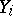of a function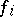is contained in the domain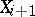of a function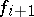, that is, if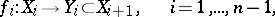then the function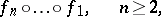defined byis called the composite function or-fold composite (or superposition) of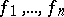. For example, every rational function in any number of variables is a composite of the four arithmetic operations, that is, of the functions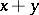,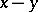,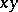, and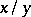.

A composite function inherits many of the properties of the functions of which it is composed. For example, the composite of continuous functions is continuous. This means that if a function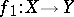is continuous at a point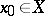, and a function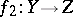is continuous at the point, then the compositeis also continuous at(here,andare, for example, topological spaces). Similarly, the composite of-fold (continuously) differentiable functions is again-fold (continuously) differentiable,. The composite of increasing (decreasing) functions is again increasing (decreasing). In composing functions quantitative properties often change: The composition of two functions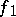and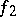satisfying a Hölder condition of certain degrees is a function satisfying the Hölder condition of degree equal to the product of the degrees of the Hölder conditions satisfied byand. Some properties of functions are not preserved under composition. For example, the composite of Riemann- or Lebesgue-integrable functions is not, in general, Riemann or Lebesgue integrable, respectively. The composite of absolutely-continuous functions can turn out not to be absolutely continuous. However, according to the results of N.K. Bari and D.E. Men'shov , composition of three absolutely-continuous functions on an interval does not lead to a wider class of functions than that obtained by composing two. Bari

proved that any continuous function on a closed interval can be written as the sum of three composites of absolutely-continuous functions, and that there are continuous functions which cannot be written as the sum of two. Moreover, every continuous function on a closed interval is the sum of two composites of functions of bounded variation. Finally,-fold composites of functions of bounded variation lead to essentially new classes of functions for everyand there are continuous functions that are not one-fold compositions of functions of bounded variation .

The concept of composition of functions constitutes the widest possible way of giving meaning to the term "representation of a function by a formula" . The problem of representing a function in terms of composites arose in connection with the discovery of formulas for the solutions of algebraic equations. Every root of an equation of degree at most four can be expressed as a composition of the four arithmetical operations and of radicals applied to the coefficients of the equation. By means of a certain substitution (called a Tschirnhausen transformation) every equation of degree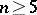can be reduced to the form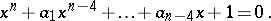Thus, every root of an equation of degreeis a function ofparameters. The problem consists of deciding whether these functions can be represented as a composition of algebraic functions in a smaller number of variables. One of the 23 problems posed by D. Hilbert at the International Congress of Mathematicians in Paris in 1900 is related to this. Namely, the thirteenth problem consists of the following (see ): Can a rootof the equation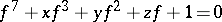(*)

be represented as a composite of certain continuous functions in two variables applied to the coefficients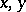and? (Note that every function in a finite number of variables is a composite of discontinuous functions in two variables.) Hilbert established the impossibility of obtaining all analytic functions in three variables by composing analytic functions in two variables. He also showed  that a solution of an equation of the nine-th degree can be written as a composite of algebraic functions in four variables (instead of in five, as immediately follows from the application of a Tschirnhausen transformation). These investigations were taken up by many mathematicians (see ).

In 1954 A.G. Vitushkin proved  that if natural numbers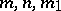, and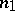satisfy the inequality, then it is possible to find an-fold differentiable function invariables that cannot be written as a composite of-fold differentiable functions in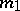variables. In particular, for everyit is possible to find a function invariables of preassigned smoothness that is not a composite of functions of a smaller number of variables and of the same smoothness. In this sense, among smooth functions of any number of variables there are functions that depend essentially on all their arguments.

In 1956, A.N. Kolmogorov showed  that every continuous function defined on an-dimensional cubeis a composite of continuous functions in three variables. Later V.I. Arnol'd reduced the number of variables from three to two. In fact, he proved  that any continuous function in three variables on a cube can be written as a composite of continuous functions in two variables (and even, more precisely, as the sum of nine functions each of which is a composite of two continuous functions in two variables). Thus, every continuous function on an-dimensional cubecan be written as a composite of continuous functions in two variables. This was the last word in refuting Hilbert's conjecture on the impossibility of representing the roots of the equation (*) in the form of composites of continuous functions in two variables. The papers of Kolmogorov and Arnol'd gave, in particular, a positive solution to the problem of representing the roots of algebraic equations of arbitrary degree as composites of continuous functions in at most two variables. For composites of analytic and algebraic functions the corresponding problem remains unsolved. It is not yet known (1987) whether or not the roots of the equation (*) are composites of analytic functions.

This series of papers is completed by the following theorem of Kolmogorov : Any continuous function invariables can be obtained by composing continuous functions in one variable and the single function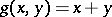in two variables. In fact, he proved that any continuous functionon an-dimensional cube can be written in the form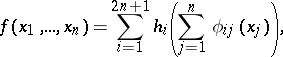where the functions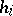and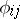are continuous, andare standard functions, that is, they do not depend on the choice of.

Vitushkin showed  that for any finite sets of continuous functions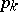and continuously-differentiable functions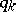invariables (;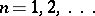), there even are analytic functions invariables that cannot be written as a composite of the form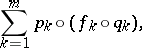where the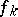are arbitrary continuous functions in one variable.

How to Cite This Entry:
Composite function. Encyclopedia of Mathematics. URL: http://encyclopediaofmath.org/index.php?title=Composite_function&oldid=12280
This article was adapted from an original article by L.D. Kudryavtsev (originator), which appeared in Encyclopedia of Mathematics - ISBN 1402006098. See original article